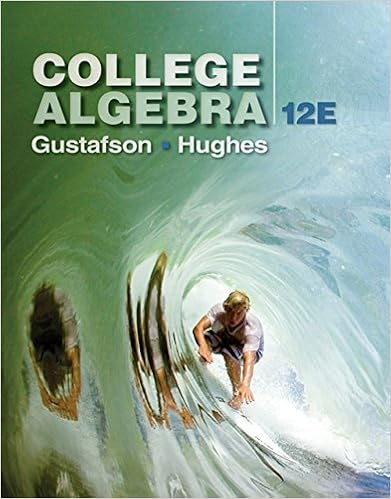# 2 - MAT 1033 COMMON FINAL REVIEW PACKET 1 Determine whether...

• Test Prep
• 21

This preview shows page 1 - 4 out of 21 pages.

##### We have textbook solutions for you!
The document you are viewing contains questions related to this textbook.The document you are viewing contains questions related to this textbook.
Chapter 2 / Exercise 55
College Algebra
Gustafson/HughesExpert Verified
MAT 1033 COMMON FINAL REVIEW PACKET 1. Determine whether the relation is a function. Give its domain and range. Is the relation represented in the table a function? box2Yes box2No The domain is { ____________ }. (Use a comma to separate answers as needed.) The range is { ____________ }. (Use a comma to separate answers as needed.) ______________________________________________________________________________ 2. Find an equation of the line that satisfies the given conditions.
The equation of the line that satisfies the given conditions is _________________. (Write an equation. Use integers or decimals for any numbers in the equation.) _______________________________________________________________________________________ 3. Match the equation with its graph (a. – f.), where m is a constant. y = mx, m > 0Choose the graph that matches the equation. box3a. box3b. box3c. box3d. box3e. box3f. x y 3 –6 3 –1 3 2 3 3
##### We have textbook solutions for you!
The document you are viewing contains questions related to this textbook.The document you are viewing contains questions related to this textbook.
Chapter 2 / Exercise 55
College Algebra
Gustafson/HughesExpert Verified
MAT 1033 Common Final Review Packet Page 24. Use the table below to evaluate the given expressions. x f(x) –4 –1 –2 0 13 2 -4 4 27 Part 1. f(–4) = ___________ Part 2. f(4) = ____________ ___________________________________________________________________________ 5. The graph to the right illustrates the average number of people living on parcels of land of various sizes in 1960. Complete parts (1) through (4). Part 1. The graph passes through the points (2, 100) and (3, 150). Discuss the meaning of these points. Choose the correct answer below. box3a. Two acres have 100 people and 3 acres have 150 people, on average. box3b. One hundred acres have exactly 2 people and 150 acres have exactly 3 people. box3c. One hundred acres have 2 people and 150 acres have 3 people, on average. box3d. Two acres have exactly 100 people and 3 acres have exactly 150 people. Part 2. Find the slope–intercept form of the line. 4 ___________________________________ Part 3. Interpret the slope as a rate of change. Choose the correct answer below. box3a. The land has exactly this many people per acre. box3b. The land has this many people per acre, on average. box3c. There are this many acres per person, on average. box3d. There are exactly this many acres per person. Part 4. Write the equation of this line as a linear function P that outputs the average number of people living on x acres of land. _________________________________ __________________________________________________________________________ 6. Find the slope–intercept form of the equation of the line with the following: x–intercept value: –6 y–intercept value: What is the slope-intercept form of the line? y = _____________________ (Use integers or simplified fractions for any numbers in the equation.) 7
MAT 1033 Common Final Review Packet Page 37. Do the following. (1) Find the slope–intercept form of the line perpendicular to the given line, passing through the given point. (2) Graph the two lines. y = –2x + 2, (–1, 1) part 1: What is the slope–intercept form of the line?(Use integers or simplified fractions for any numbers in the equation.) part 2: Choose the graph below that shows in bold and also the perpendicular line. box3
d. __________________________________________________________________________________
•••